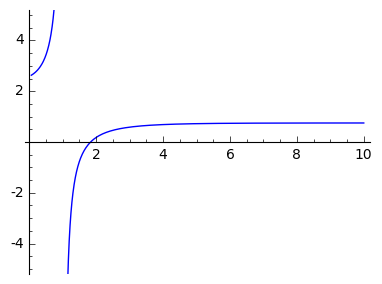# Solving zeta function equation numerically

I'm trying to solve the equation $$\zeta'(x)/\zeta(x) = - 3/4$$ numerically. I'm expecting/hoping for an answer between 1 and 10.

I tried

$\texttt{ find_root(diff(zeta(x))/zeta(x) + 0.75, 2, 40)}$,

but this returns 0.0. I don't know what that ratio of zeta functions looks like, so it's possible there isn't a root, but I don't see why I'm getting an answer outside the specified interval.

Can anyone help find the true root? Thanks.

edit retag close merge delete

What version of Sage are you using? I get the following in Sage 7.2:

sage: find_root(diff(zeta(x))/zeta(x)+.75, 2, 40)
Traceback (most recent call last):
...
RuntimeError: f appears to have no zero on the interval


Sort by » oldest newest most voted

If you plot your function first, for example with

f = diff(zeta(x))/zeta(x)+.75
plot(f,0,10,ymin=-5,ymax=5,detect_poles=True,figsize=[4,3])


you can see approximately where the zero is:You just need to change the range over which you're searching:

find_root(f,0,10)
1.8333259941894324


Here's a link to live example.

more